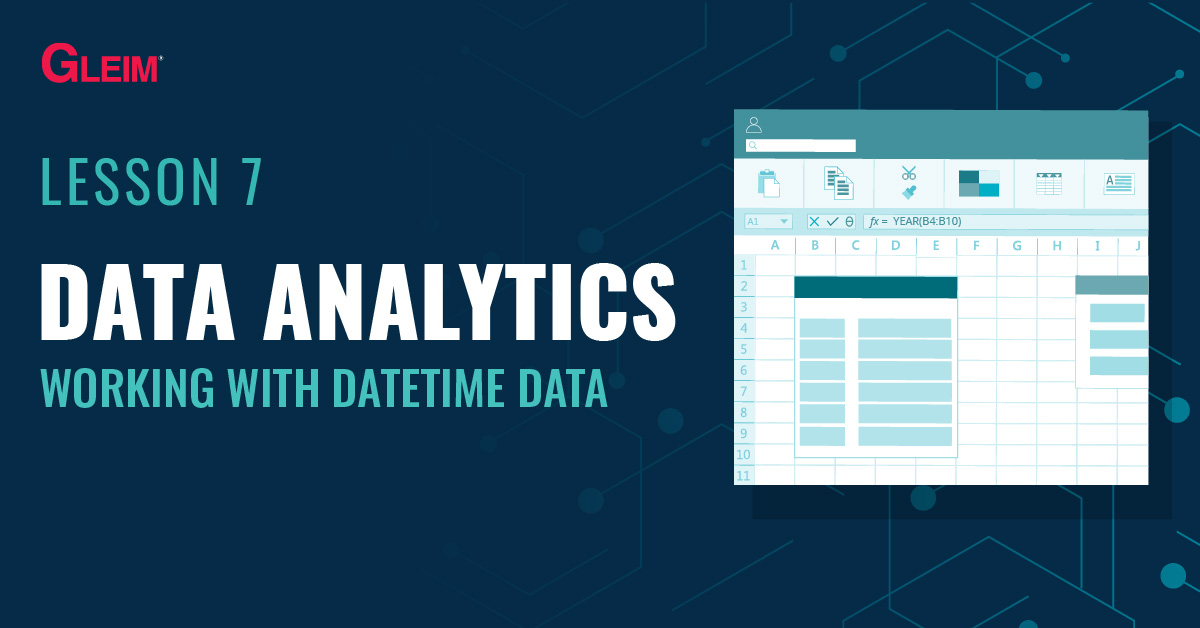# Excel Functions: Working with Datetime DataUnlike numerical values, datetime data generally cannot be summarized by a statistic such as the sum or average. Yet, insights from datetime data can be drawn by aggregating them into specific time intervals and investigating the trends across those intervals. For example, aggregating data by month can highlight seasonal trends throughout the year. This blog introduces a list of common functions to work with datetime data.

## Extracting Components from a Date

The following functions extract the individual components of a datetime entry:

• =year(serial_number)
• =month(serial_number)
• =day(serial_number)
• =hour(serial_number)
• =minute(serial_number)
• =second(serial_number)

Assume the following dataset [you may paste it into Excel]:

 A 1 Date 2 1/8/2020 3 2020-09-12 4 12/15/18 5 April 20, 2020 6 11/29/17 8:12 AM

Despite the different formats, all entries are datetime data.

Examples:
The formula = year(A2) returns the result of 2020.
The formula = month(A3) returns the result of 9.
The formula = day(A4) returns the result of 15.
The formula = hour(A5) returns the result of 0 [4/20/2020 00:00:00].
The formula = minute(A6) returns the result of 12 [08:12].

## Extracting the Day of the Week

### weekday   function

The   weekday   function finds the total of the numerical values specified as the arguments and has the following syntax:

= weekday(serial_number,[return_type])
Note: The return type argument specifies how the returned value denotes the day of the week. There are two options (1 or 2). If this argument is blank the formula will default to 1.

Return Type 1 (default) Return Type 2

Sunday: 1

Saturday: 7

Monday: 1

Sunday: 7

Example:
The formula = weekday(“1/1/2020”,1) returns the number 4, indicating that 1/1/2020 falls on the fourth day of the week starting on Sunday (i.e., Wednesday).

## Finding the End or Start of a Month

### eomonth   function

The  eomonth   function returns the end of (last day of) the month a certain number of months before or after a start date. It has the following syntax:

= eomonth(start_date,months)
Assume the following dataset [you may paste it into Excel]:

 A 1 Date 2 1/8/2020 3 2020-09-12 4 12/15/18 5 April 20, 2020 6 11/29/17 8:12 AM

Example 1:
The formula = eomonth(A2, 0) returns 1/31/2020, the last day of the month that is 0 months after 1/8/2020.

The formula = eomonth(A3, 2) returns 11/30/2020, the last day of the month that is 2 months after 9/12/2020.

Example 2:
The formula = eomonth(A2,-1) + 1 returns 1/1/2020, the first day of the month (the day after the last day of the previous month).

## Difference between Two Dates

### days   function

The  days   function returns the difference in days between a start date and an end date. It has the following syntax:

= days(end_date,start_date)
Assume the following dataset [you may paste it into Excel]:

 A 1 Date 2 1/8/2020 3 2020-09-12 4 12/15/18 5 April 20, 2020 6 11/29/17 8:12 AM

Example:
The formula = days(eomonth(A2, 0), A2) returns 23, the difference in days between 1/8/2020 and 1/31/2020 (the end of the month).

### datedif   function

The  datedif   function returns the difference in time (complete year, complete month, complete day) between a start date and an end date. It has the following syntax:

= datedif(start_date,end_date,unit)
Note: The unit can be year(“y”), month(“m”), or day(“d”).

Assume the following dataset [you may paste it into Excel]:

 A 1 Date 2 1/8/2020 3 2020-09-12 4 12/15/18 5 April 20, 2020 6 11/29/17 8:12 AM

Example 1:
The formula = datedif(A2, A3, “d”) returns 248, the difference in days between 1/8/2020 and 9/12/2020.

Example 2:
The formula = datedif(A4, A3, “m”) returns 8, the difference in months between 12/15/2019 and 09/12/2020 [The formula returns 8 (complete months) instead of 9 (December to September)].

## Learning Excel Data Analytics with Gleim

At Gleim, we know learning data analytics is vital for future accounting professionals. That is why we are offering these series of data analytics blogs and continually updating all of our CPA, CMA, and CIA Review materials with the necessary information you need to pass your exams.

We’ll continue our weekly blog series. Check back regularly for exam news and study tips!

Python Lessons
Python Basics
Conditional Statements
Loops
Functions and Modules
Numerical Python (NumPy)
Pandas Basics
Pandas Data Capture and Cleansing
Merging and Grouping
Manipulating Text and Datetime Data
Visualization
Web Scraping
Errors and Exceptions